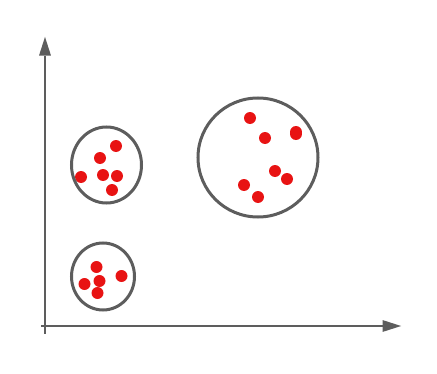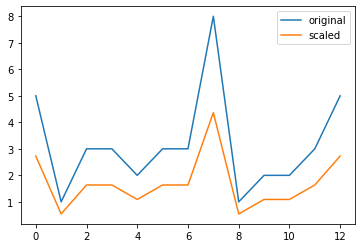top of page

# Unsupervised Learning: Basics of Clustering## What is unsupervised learning?

• A group of machine learning algorithms that find patterns in data

• Data for algorithms has not label, classified or characterized

• The objective of the algorithm is to interpret any structure in the data

• Common unsupervised learning algorithms: clustering, neural networks, anomaly detection

## What is clustering?

• The process of grouping items with similar characteristics

• Items in groups similar to each other than in other groups

• Example: distance between points on a 2D plane

## Plotting data for clustering - Pokemon sightings

```
from matplotlib import pyplot as plt
x_coordinates = [80, 93, 86, 98, 86, 9, 15, 3, 10, 20, 44,
56, 49, 62, 44]
y_coordinates = [87, 96, 95, 92, 92, 57, 49, 47, 59, 55, 25,
2, 10, 24, 10]
plt.scatter(x_coordinates, y_coordinates)
plt.show()```Data are separated into three parts!!!

## What is a cluster?

• Group of items with similar characteristics

• Google News: articles where similar words and word associations appear together

• Customer Segments

## Clustering algorithms

• Hierarchical clustering

• K means clustering

• Other clustering algorithms: DBSCAN, Gaussian Methods

## Hierarchical clustering in SciPy

```from scipy.cluster.hierarchy import linkage, fcluster
from matplotlib import pyplot as plt
import seaborn as sns, pandas as pd

x_coordinates = [80.1, 93.1, 86.6, 98.5, 86.4, 9.5, 15.2,
3.4, 10.4, 20.3, 44.2, 56.8, 49.2, 62.5, 44.0]
y_coordinates = [87.2, 96.1, 95.6, 92.4, 92.4, 57.7, 49.4,
47.3, 59.1, 55.5, 25.6, 2.1, 10.9, 24.1, 10.3]

df = pd.DataFrame({'x_coordinate': x_coordinates,
'y_coordinate': y_coordinates})

Z = linkage(df, 'ward') # ward: based on the sum of squares
df['cluster_labels'] = fcluster(Z, 3, criterion='maxclust')

sns.scatterplot(x='x_coordinate', y='y_coordinate', hue='cluster_labels', data = df)
plt.show()```## K-means clustering in SciPy

```from scipy.cluster.vq import kmeans, vq
from matplotlib import pyplot as plt
import seaborn as sns, pandas as pd
import random
random.seed((1000,2000))

x_coordinates = [80.1, 93.1, 86.6, 98.5, 86.4, 9.5, 15.2,
3.4, 10.4, 20.3, 44.2, 56.8, 49.2, 62.5, 44.0]
y_coordinates = [87.2, 96.1, 95.6, 92.4, 92.4, 57.7, 49.4,
47.3, 59.1, 55.5, 25.6, 2.1, 10.9, 24.1, 10.3]

df = pd.DataFrame({'x_coordinate': x_coordinates,
'y_coordinate': y_coordinates})

centroids,_ = kmeans(df, 3)
df['cluster_labels'], _ = vq(df, centroids) # centroid: based on the geometric mean of all objects

sns.scatterplot(x='x_coordinate', y='y_coordinate',
hue='cluster_labels', data = df)
plt.show()```## Why do we need to prepare data for clustering?

• Variables have incomparable units (product dimensions in cm, price in \$)

• Variables with the same units have vastly different scales and variances (expenditures oncereals, travel)

• Data in raw form may lead to bias in clustering

• Clusters may be heavily dependent on one variable

• Solution: normalization of individual variables

## Normalization of data

Normalization: the process of rescaling data to a standard deviation of 1

```from scipy.cluster.vq import whiten
data = [5, 1, 3, 3, 2, 3, 3, 8, 1, 2, 2, 3, 5]
scaled_data = whiten(data)
print(scaled_data)```
```[2.72733941 0.54546788 1.63640365 1.63640365 1.09093577 1.63640365
1.63640365 4.36374306 0.54546788 1.09093577 1.09093577 1.63640365
2.72733941]```
```# Import plotting library
from matplotlib import pyplot as plt

# Initialize original, scaled data
plt.plot(data,label="original")
plt.plot(scaled_data,label="scaled")# Show legend and display
plot

plt.legend()
plt.show()```